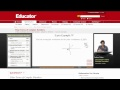### Total 17 Videos found in Category "Pre-Calculus"

Title
 1Pre-Calculus: Algebraic Representation of Vectors 2Pre-Calculus: Complex Numbers 3Pre-Calculus: de Moivre Formula 4Pre-Calculus: Expected Value (Probability) 5Pre-Calculus: Fundamental Theorem of Algebra 6Pre-Calculus: Gauss Jordan Method 7Pre-Calculus: Geometric Vectors 8Pre-Calculus: Graphing Polar Coordinates 9Pre-Calculus: Mathematical Induction 10Pre-Calculus: Parametric Equations to Model Motion 11Pre-Calculus: Polar Coordinates 12Pre-Calculus: Polar Forms of Complex Numbers 13Pre-Calculus: Products and Quotients of Complex Numbers in Polar Form 14Pre-Calculus: Rational Functions 15Pre-Calculus: Simplifying Complex Numbers 16Pre-Calculus: Vectors and Parametric Equations 17Pre-Calculus: Vectors in 3D Space

Say and share some thing about these videos...